# Hartogs theorem

(diff) ← Older revision | Latest revision (diff) | Newer revision → (diff)
Hartogs' basic (principal, fundamental) theorem: If the function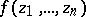, defined in a domain, is holomorphic at every point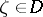with respect to each variable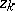(for fixed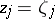,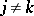,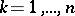), then it is holomorphic inwith respect to all variables. There exist many generalizations of this theorem to include cases when some of the variables are real, or when not all points of the domainare used or when some singularities ofare permitted. For example: a) if a function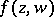,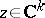,, defined in the domain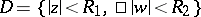, is holomorphic in the domain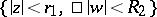,, and is holomorphic in the ball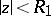for any given,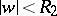, then it is holomorphic in; b) if a functiondefined onwith values in the extended complex plane is rational with respect to each variable, then it is a rational function.
Hartogs' extension theorem: Let a domain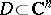have the form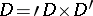, where,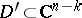, and let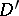be bounded. Then any functionthat is holomorphic in a neighbourhood of the set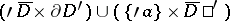,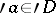, can be holomorphically extended to.
Hartogs' theorem is also taken to be the theorem on the removability of compact singularities (if); it is also known as the Osgood–Brown theorem .
The name Hartogs' theorem is also given to theorems on the continuous distribution of singular points if, on the analyticity of the set of singular points, and the theorem of uniform boundedness of a sequence of pointwise-bounded subharmonic functions.